``gene_data = read.csv("gene_expression_data.csv")``

### Help

To check how the read.csv function works check the read.csv page by typing ?read.csv in the console.

``?read.csv``

This is just a simple description. The help page has much more useful information!

``````read.table(file, header = FALSE, sep = "", quote = "\"'",
dec = ".", numerals = c("allow.loss", "warn.loss", "no.loss"),
row.names, col.names, as.is = !stringsAsFactors,
na.strings = "NA", colClasses = NA, nrows = -1,
skip = 0, check.names = TRUE, fill = !blank.lines.skip,
strip.white = FALSE, blank.lines.skip = TRUE,
comment.char = "#",
allowEscapes = FALSE, flush = FALSE,
stringsAsFactors = default.stringsAsFactors(),
fileEncoding = "", encoding = "unknown", text, skipNul = FALSE)

dec = ".", fill = TRUE, comment.char = "", ...)

dec = ",", fill = TRUE, comment.char = "", ...)

dec = ".", fill = TRUE, comment.char = "", ...)

dec = ",", fill = TRUE, comment.char = "", ...)``````

### Check what is inside

We can quickly check what is inside this object with a few commands.

``dim(gene_data)     # gives us the dimensions of the table``
``##  305   4``
``head(gene_data)    # shows us the first 6 rows of the table``
``````##           X     geneA     geneB   cell_type
## 1 FIBRO-9DW 0.7542634 0.9423952 FIBROBLASTS
## 2 FIBRO-TPA 0.7476058 0.9396038 FIBROBLASTS
## 3 FIBRO-TYL 0.7476938 0.9457088 FIBROBLASTS
## 4 FIBRO-4LI 0.7667875 0.9727001 FIBROBLASTS
## 5 FIBRO-7CJ 0.7712265 0.9645454 FIBROBLASTS
## 6 FIBRO-50S 0.7625049 0.9724643 FIBROBLASTS``````
``summary(gene_data) # shows us information about the different columns of the table``
``````##          X           geneA            geneB              cell_type
##  BLOOD-05K:  1   Min.   :0.7318   Min.   :0.9116   BLOOD.CELLS:184
##  BLOOD-09X:  1   1st Qu.:0.7630   1st Qu.:0.9560   FIBROBLASTS:108
##  BLOOD-0I1:  1   Median :1.3015   Median :0.9798   IPSC       : 13
##  BLOOD-0L7:  1   Mean   :1.0982   Mean   :0.9822
##  BLOOD-0UW:  1   3rd Qu.:1.3158   3rd Qu.:0.9926
##  BLOOD-0WF:  1   Max.   :1.3348   Max.   :1.2552
##  (Other)  :299``````

With the previous commands we can already say quite a few things about the data we have loaded. It has 305 rows and 4 columns. The 1st column is an experiment identifier, the 2nd and 3rd columns is the gene expression information about two genes (geneA and geneB). Finally, the 4th column is named “cell_type”, which contains the cell type associated with each different sample.

We can use the command “table” on the column “cell_type” of the data we loaded to obtain a count of each of the different cell types present in this data in an easy to read form.

If you remember, from the previous exercises and the lecture, this is done with “”. Further since we are looking at some sort of matrix and we want a column, we know that we should place the name of the column after a comma (data[,“name_of_column”]).

``````count_cell_types = table(gene_data[,"cell_type"])
count_cell_types``````
``````##
## BLOOD.CELLS FIBROBLASTS        IPSC
##         184         108          13``````

### Help

For detailed help use ?NAME_OF_FUNCTION.

``````?dim
?table
?summary``````

dim

``````dim(x)
dim(x) <- value``````

``````head(x, ...)
## Default S3 method:
## S3 method for class 'data.frame'
## S3 method for class 'matrix'
## S3 method for class 'ftable'
## S3 method for class 'table'
## S3 method for class 'function'

tail(x, ...)
## Default S3 method:
tail(x, n = 6L, ...)
## S3 method for class 'data.frame'
tail(x, n = 6L, ...)
## S3 method for class 'matrix'
tail(x, n = 6L, addrownums = TRUE, ...)
## S3 method for class 'ftable'
tail(x, n = 6L, addrownums = FALSE, ...)
## S3 method for class 'table'
tail(x, n = 6L, addrownums = TRUE, ...)
## S3 method for class 'function'
tail(x, n = 6L, ...)``````

table

``````table(...,
exclude = if (useNA == "no") c(NA, NaN),
useNA = c("no", "ifany", "always"),
dnn = list.names(...), deparse.level = 1)

as.table(x, ...)
is.table(x)

## S3 method for class 'table'
as.data.frame(x, row.names = NULL, ...,
responseName = "Freq", stringsAsFactors = TRUE,
sep = "", base = list(LETTERS))``````

summary

``````summary(object, ...)

## Default S3 method:
summary(object, ..., digits, quantile.type = 7)
## S3 method for class 'data.frame'
summary(object, maxsum = 7,
digits = max(3, getOption("digits")-3), ...)

## S3 method for class 'factor'
summary(object, maxsum = 100, ...)

## S3 method for class 'matrix'
summary(object, ...)

## S3 method for class 'summaryDefault'
format(x, digits = max(3L, getOption("digits") - 3L), ...)
## S3 method for class 'summaryDefault'
print(x, digits = max(3L, getOption("digits") - 3L), ...)``````

With the information gathered through the few commands we ran, we can say that:

• the table has the gene expression values for genes A and B for 305 different samples from 3 different cell types.
• there are 184 blood cells samples, 108 fibroblasts and 13 IPSCs in this dataset.

### Plotting

There are several different functions that you can use to analyse this data. For example we can use the count data that we generated above with the table function to quickly generate a pie chart or bar plot with the number of samples per cell type in our data.

``pie(count_cell_types)``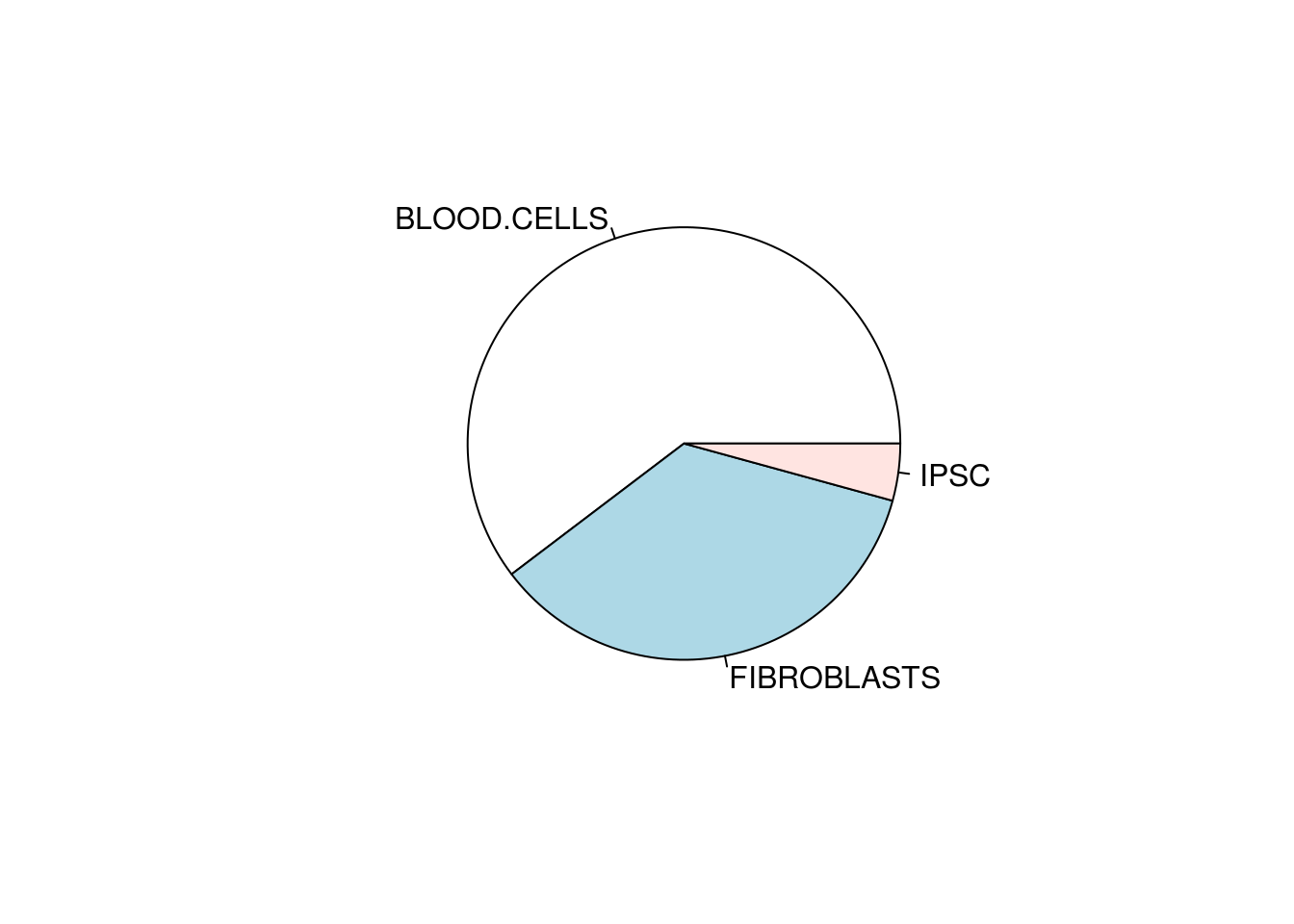``barplot(count_cell_types)``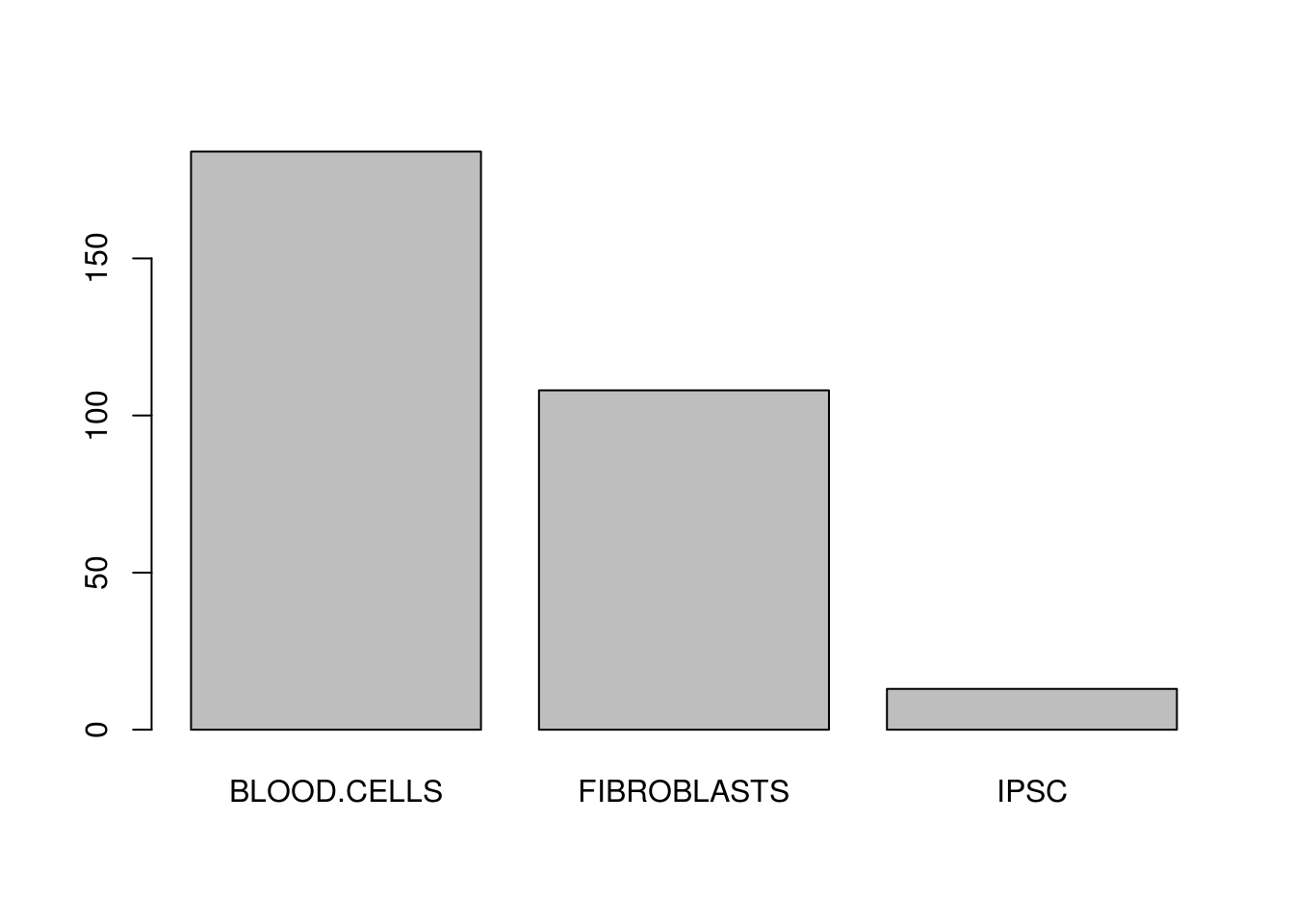These are fine but we can define our own colours that we can use here and throughout the whole exercise to keep the plots consistent with each other. R has a number of predefined colours that you can call by name (e.g. “red”,“black”,“green”) and which you can check in this link. You can also use a hexadecimal colour code, commonly called hexcolor, to define over 16 million colours. In “hexcolor”, red can be “#ff0000”, black is “#000000” and green can be “#42853c”. Hexcolour codes always start with a “#” symbol followed by 6 alfanumerical characters.

In this exercise we will use the colors pre-defined in R but feel free to change these to colours you like. Lets define cell_colours and use it throughout the exercise.

``cell_colours = c("red", "blue", "green")``

Lets repeat the previous plots but with colours.

``pie(count_cell_types, col=cell_colours)     # the col argument defines the color``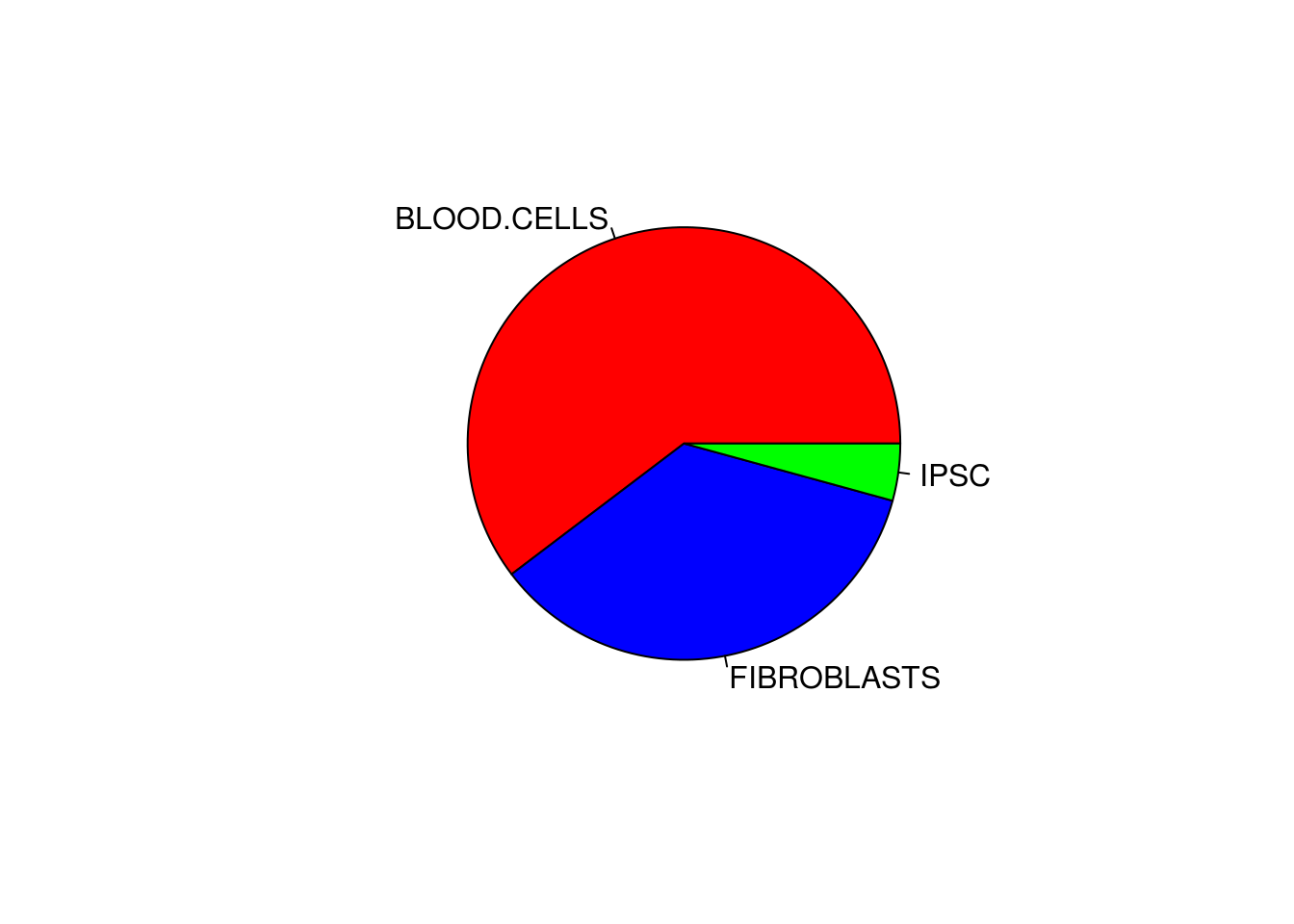``barplot(count_cell_types, col=cell_colours) # the col argument defines the color``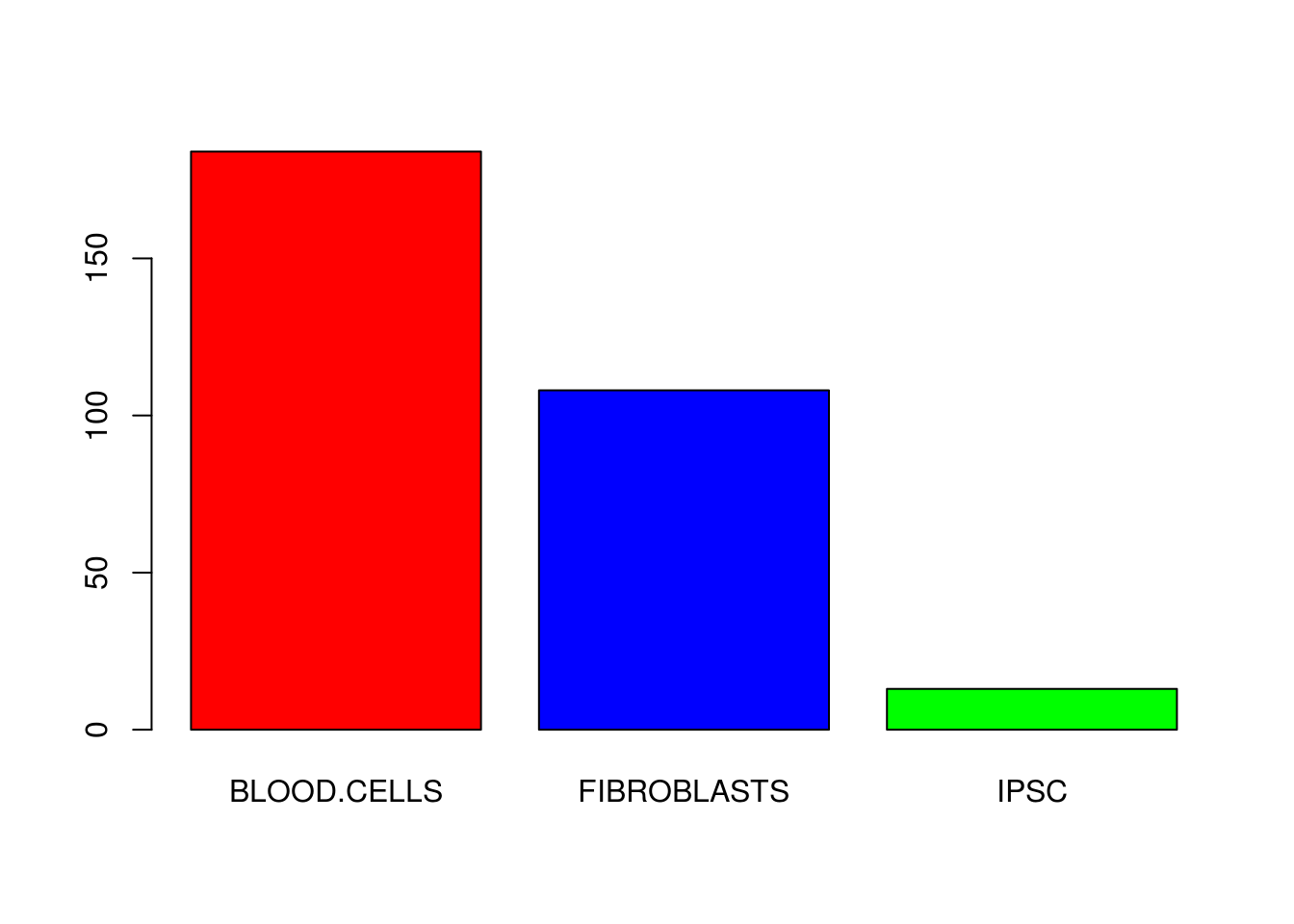A scatter plot, where we show the expression of the two genes for all samples, provides the best vizualisation for this dataset. To do this, we can use the function plot. It has many arguments and you should check its help page. To start with we will just use the x and y arguments.

``````plot(
x=gene_data[,"geneA"], # data in the x axis
y=gene_data[,"geneB"]  # data in the y axis
)``````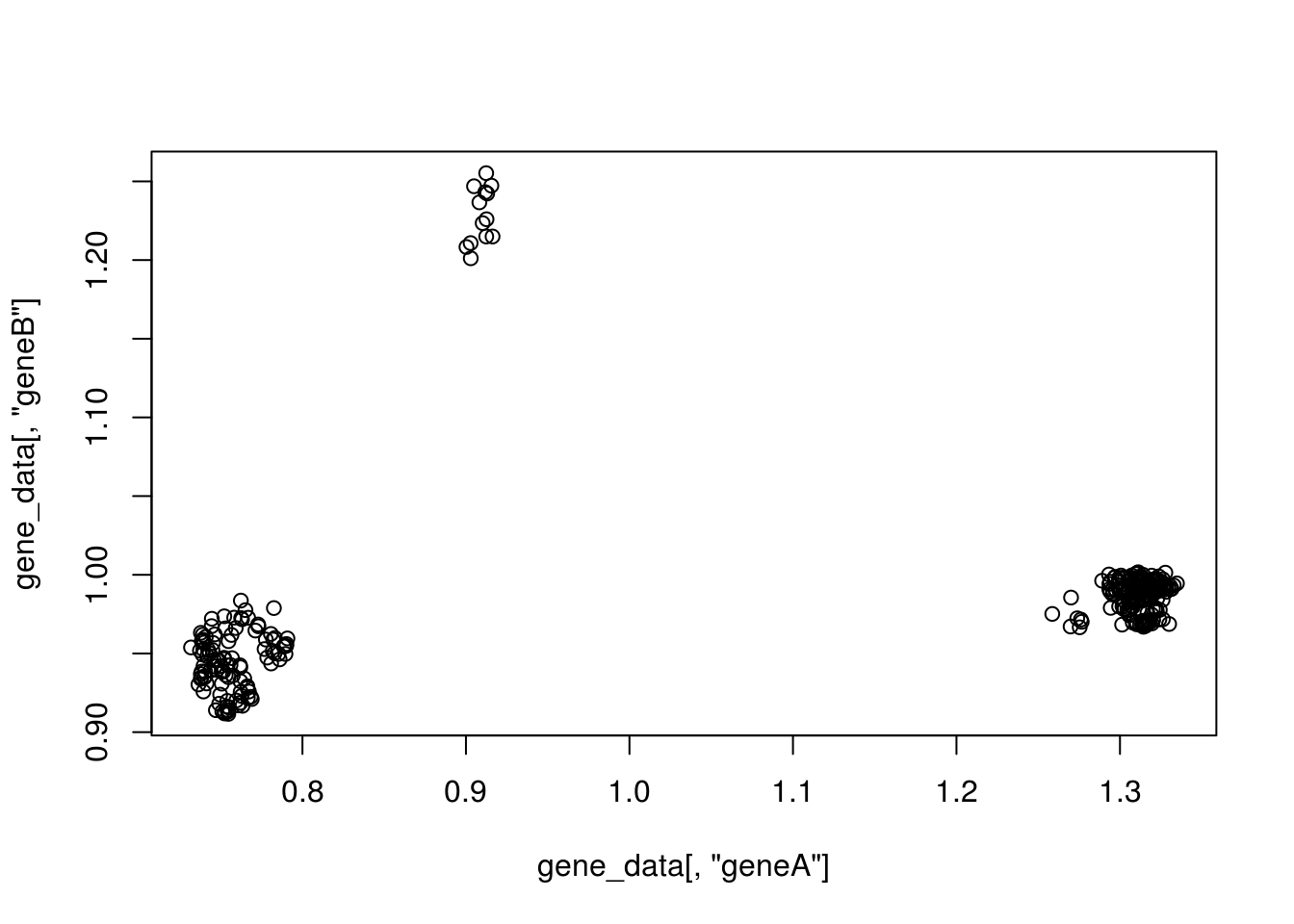### Help

For more details do ?plot, ?pie, ?barplot.

``plot(x, y, ...)``
``````pie(x, labels = names(x), edges = 200, radius = 0.8,
clockwise = FALSE, init.angle = if(clockwise) 90 else 0,
density = NULL, angle = 45, col = NULL, border = NULL,
lty = NULL, main = NULL, ...)``````
``````barplot(height, ...)

## Default S3 method:
barplot(height, width = 1, space = NULL,
names.arg = NULL, legend.text = NULL, beside = FALSE,
horiz = FALSE, density = NULL, angle = 45,
col = NULL, border = par("fg"),
main = NULL, sub = NULL, xlab = NULL, ylab = NULL,
xlim = NULL, ylim = NULL, xpd = TRUE, log = "",
axes = TRUE, axisnames = TRUE,
cex.axis = par("cex.axis"), cex.names = par("cex.axis"),
inside = TRUE, plot = TRUE, axis.lty = 0, offset = 0,
add = FALSE, ann = !add && par("ann"), args.legend = NULL, ...)

## S3 method for class 'formula'
barplot(formula, data, subset, na.action,
horiz = FALSE, xlab = NULL, ylab = NULL, ...)``````

This shows that samples group within the expression space, but we do not have information about the cell types. We can add visual queues to vizualise the distribution of distinct cell types by using colour.

We want to colour each sample by its cell type.
To do this we will use the “cell_type” column in our table.
We will save this column to a variable and subsequently transform it to a factor.
Factors are just easier to work with in this context.

``````cell_type_data = as.factor(gene_data[,"cell_type"])

plot(
x=gene_data[,"geneA"], # data in the x axis
y=gene_data[,"geneB"], # data in the y axis
col=cell_type_data     # colour
)``````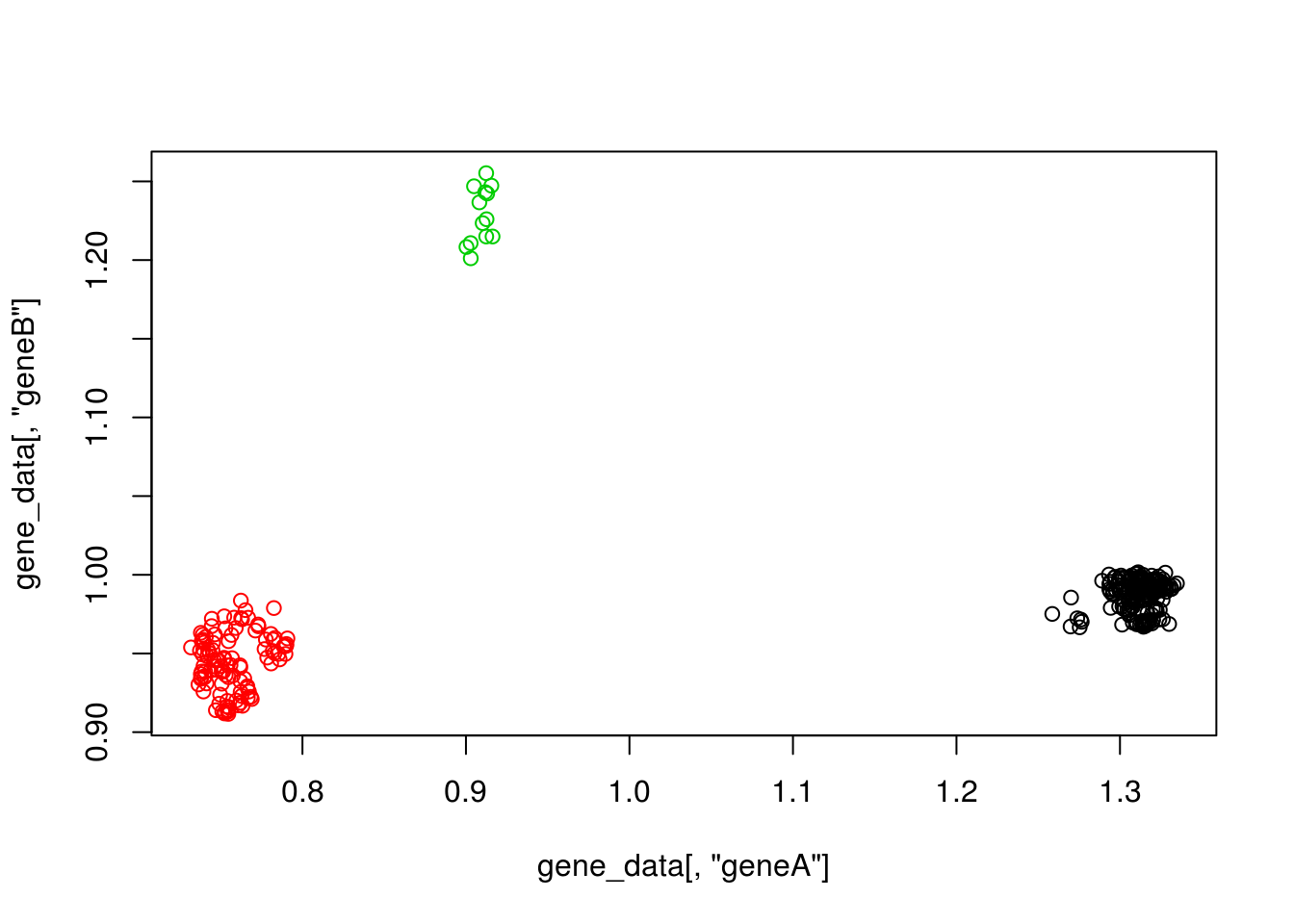Because of how factors work we can use them to subset the colour vector we created (cell_colours) with the categories of the factor itself (the different cell types). This makes it so that each of the cell types has the colour we want for it.

``````plot(
x=gene_data[,"geneA"], # data in the x axis
y=gene_data[,"geneB"], # data in the y axis
col=cell_colours[cell_type_data]     # colour
)``````